﻿ 桩板墙在深路堑中的应用分析与优化设计

# 桩板墙在深路堑中的应用分析与优化设计Analysis and Optimization Design of Pile Slab Wall on Deep Cut

Abstract: With the development of society, some deep cutting projects have appeared in the construction of railway and highway projects. In deep cutting engineering, excavation will lead to the redistribution of initial ground stress and break the original ground stress balance, thus causing a series of stability problems of engineering slope. Therefore, it is very important to choose the proper structure in the engineering. In this paper, Midas GTS finite element software is used to carry out numerical simulation stress analysis on a pile slab wall in a deep cutting, and a comparative study is conducted on the pile slab wall under different working conditions to optimize the design. Finally, its stress characteristics are obtained. The optimal scheme is 20 m pile, 2 × 2.5 m section size and 6 m pile spacing.

1. 引言

Begemann和De Leeuw  定义了压力法，并且给出了压力的计算方法：在计算从地面来的荷载作用在桩上的力时，可以将桩看成是刚性桩，当计算水平位移和应力分布时，可以用弹性法，桩则可以看作等值桩板墙。

2. 桩板墙理论计算

2.1. 土压力分类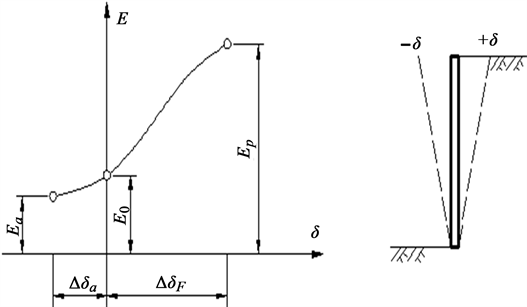Figure 1. Diagram of displacement of retaining wall and earth pressure

2.2. 桩身内力计算

① 当受到的荷载为均布荷载时(k法)，桩顶的挠曲微分方程为

$EI\frac{{\text{d}}^{4}x}{\text{d}{y}^{4}}+xK{B}_{p}=0$ (1)

② 当所受荷载为线性分布荷载时(m法)，桩的挠曲微分方程为

$EI\frac{{\text{d}}^{4}x}{\text{d}{y}^{4}}+myx{B}_{p}=0$ (2)

3. 三维模型的建立及参数选取

3.1. 本构模型和单元体的选择

1) 本构模型的选择

2) 单元体的选择

3.2. 几何模型的建立

1) 模型尺寸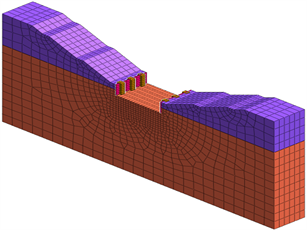Figure 2. Three-dimensional finite element model of cutting pile wall

2) 土体参数Table 1. The value of material related parameterst

3.3. 开挖过程模拟

① 初始应力阶段；

② 开挖第一层土；

③ 开挖第二层土；

④ 开挖第三层土；

⑤ 挖孔建桩；

⑥ 安装挡土板。

4. 桩板墙三维数值模拟结果分析

4.1. 整体应力

4.1.1. 整体水平应力Figure 3. Horizontal displacement contour map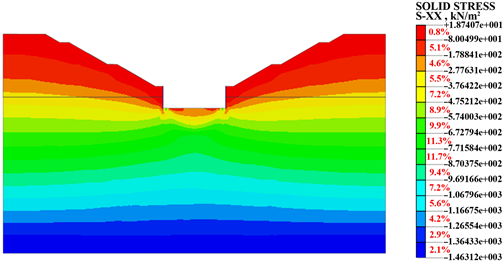Figure 4. Curve: system result of standard experiment

4.1.2. 整体竖向应力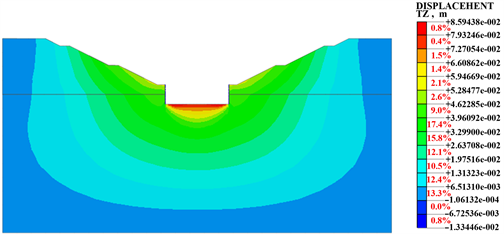Figure 5. Vertical displacement contour map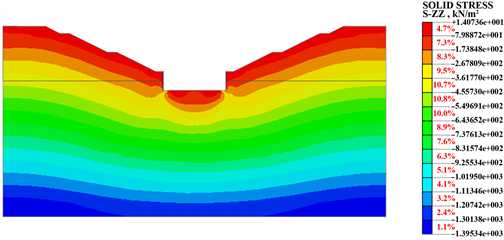Figure 6. Vertical stress equivalent nephogram

4.2. 桩身应力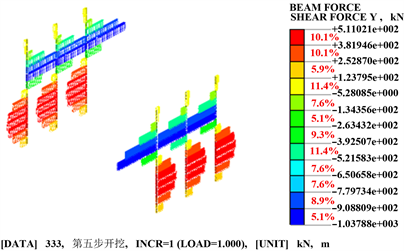Figure 7. Pile shear diagram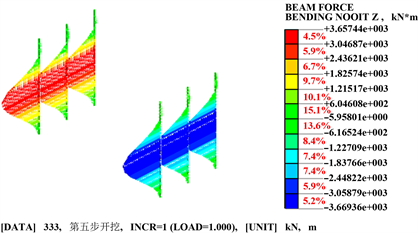Figure 8. Pile bending moment diagram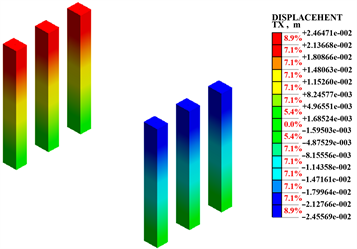Figure 9. Pile displacement diagram

4.3. 桩身长度长对比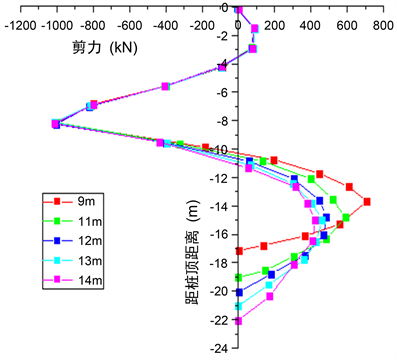Figure 10. Comparison diagram of shear force under different pile anchorage depths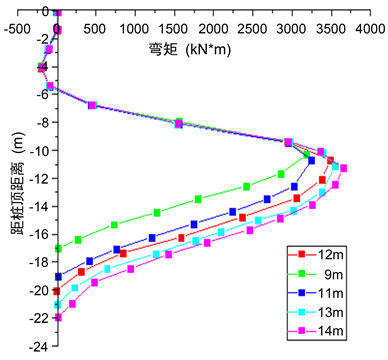Figure 11. Comparison diagram of bending moment at different pile anchorage depthsTable 2. Calculation results of pile stress of different pile lengtht

4.4. 桩截面尺寸对比Figure 12. Comparison diagram of section shear force under different section sizes of pile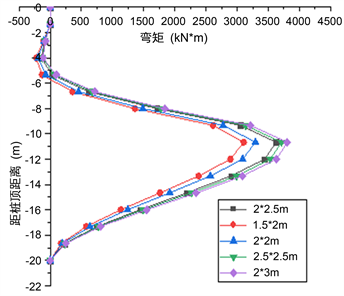Figure 13. Comparison diagram of bending moment under different section sizes of pileTable 3. Calculation results of pile stress of different pile sections

4.5. 桩间距对比Figure 14. Comparison diagram of shear force under different pile spacing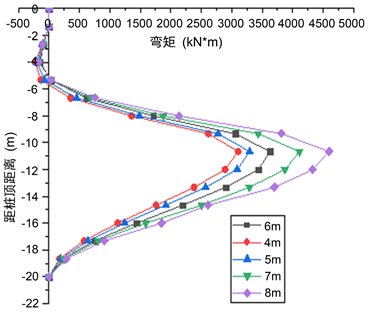Figure 15. Comparison diagram of bending moment under different pile spacingTable 4. Calculation results of pile stress at different pile spacing

5. 结论

1) 整个结构的水平应力分布较为均匀，水平位移倾向于桩板墙内侧方向，水平最大位移出现在桩顶。竖向应力分布也较均匀，竖向位移的最大值出现在路堑底部中心位置。竖向应力与水平应力的变化趋势均为随地层深度的增加而递增。路堑两侧的桩体的弯矩极值均在锚固段顶部，桩体的最大位移出现在桩顶且满足规范要求，此桩板墙结构满足设计要求不会发生失稳现象。

2) 不同的桩长截面尺寸以及桩间距下，桩身的内力以及位移均有所变化，但在到达某一数值后变化幅度明显减小说明在桩身的锚固段达到一定的长度后，对桩身内力逐渐没有了影响。在考虑地质概况，施工难度以及经济安全可行性的条件下选择桩身20 m，截面尺寸2 × 2.5 m，桩间距6 m作为最优方案。

 袁正龙. 桩板墙内侧土压力与工程特性研究[J]. 施工技术, 2019: 13-16.

 罗渝, 许强, 何思明, 等. 桩板墙结构加固边坡的稳定性分析[J]. 西南交通大学学报, 2014, 49(6): 1-6.

 李治. Midas/GTS在岩土工程中的应用[M]. 北京: 中国建筑工业出版社, 2012: 2413-2413.

 东南大学, 浙江大学, 湖南大学等. 土力学[M]. 北京: 中国建筑出版社, 2001: 72-78.

 李海光. 新型支挡结构设计与工程实例[M]. 北京: 人民交通出版社, 2004: 145-148.

 刘兴远. 边坡工程[M]. 北京: 中国建筑工程出版社, 2007: 121-126.

 Begemann, H.K.S. and De Leeuw, E.H. (1972) Horizontal Earth Pressures on Foundation Piles as a Result of Nearby Soil Fills. Proceedings of the 5th International Conference on Soil Mechanics and Foundation Engineering, Madrid, 17-22 July 1961, 3-9.

 许宏发, 吴华杰. 桩土接触单元参数分析[J]. 探矿工程, 2002(5): 10-12.

 铁道第二勘察设计院. TB 10025-2006铁路路基支挡结构设计规范[S]. 北京: 中国铁道出版社, 2006．

 巨能攀, 黄润秋, 涂国祥. 桩板墙桩土作用机理有限元分析[J]. 成都理工大学学报(自然科学版), 2006(4): 365-370.

Top